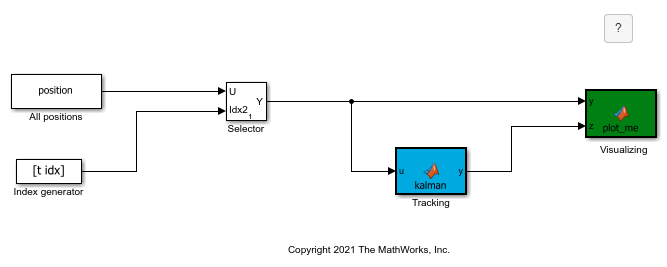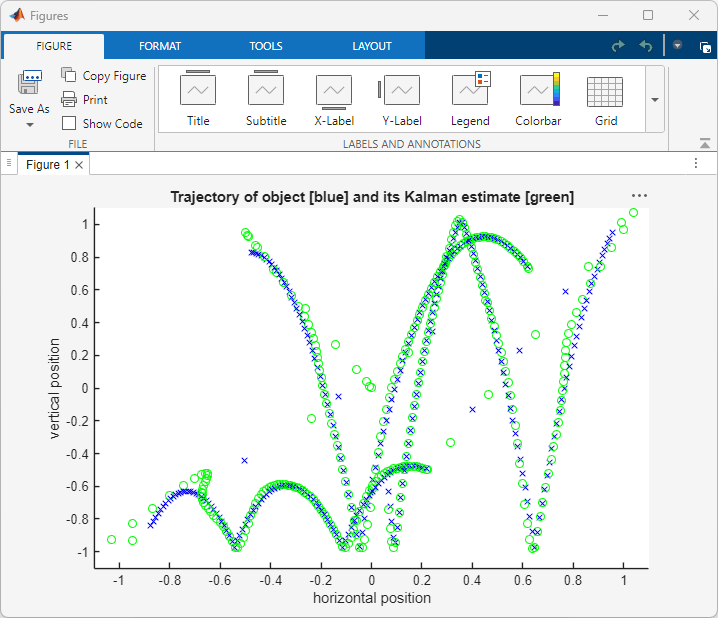# Call MATLAB Function Files in MATLAB Function Blocks

The example model uses a MATLAB Function block to estimate the location of a moving object by calling a MATLAB® function file on the path. The model then plots this prediction data against the actual location of the object with another MATLAB Function block. The example then allows you to generate code from the MATLAB Function block that calls the MATLAB function file.### Simulate the Model

The model takes the position data of an object stored in a MAT-file and runs the data through a tracking algorithm. The MATLAB Function block labeled `Tracking` calls a separate MATLAB function file, `ex_kalman_f.m`, to execute the tracking algorithm. In the model, double-click the `Tracking` block to see the code that calls `ex_kalman_f.m`.

```function y = kalman(u) y = ex_kalman_f(u); end ```

Open `ex_kalman_f.m` to view the algorithm. `ex_kalman_f.m` uses a Kalman filter algorithm to track the location of the object. The algorithm uses two persistent variables. `x_est` stores the state between time steps and `p_ext` stores the covariance. The algorithm uses these persistent variables to calculate the estimated location of the object at every time step as the output `y`.

```function y = ex_kalman_f(z) %#codegen ```
```% Initialize state transition matrix dt = 1; A = [ 1 0 dt 0 0 0;... 0 1 0 dt 0 0;... 0 0 1 0 dt 0;... 0 0 0 1 0 dt;... 0 0 0 0 1 0 ;... 0 0 0 0 0 1 ]; ```
```% Measurement matrix H = [ 1 0 0 0 0 0; 0 1 0 0 0 0 ]; Q = eye(6); R = 1000 * eye(2); ```
```% Initial conditions persistent x_est p_est if isempty(x_est) x_est = zeros(6, 1); p_est = zeros(6, 6); end % Predicted state and covariance x_prd = A * x_est; p_prd = A * p_est * A' + Q; ```
```% Estimation S = H * p_prd' * H' + R; B = H * p_prd'; klm_gain = (S \ B)'; ```
```% Estimated state and covariance x_est = x_prd + klm_gain * (z - H * x_prd); p_est = p_prd - klm_gain * H * p_prd; ```
```% Compute the estimated measurements y = H * x_est; end ```

MATLAB Function block code, and the function files the block calls, must be supported for code generation. Unlike the MATLAB Function block, MATLAB files called by the MATLAB Function block require the `%#codegen` directive to find code violations that would result in errors during code generation. In this example, `ex_kalman_f.m` does not have any violations.

After Simulink® calculates the estimated location of the object, the `Visualizing` block plots the actual and estimated locations of the object by using the MATLAB `plot` function.

```function plot_me(y,z) ```
```persistent h if isempty(h) h = figure; hold; end ```
```N = size(z,2); title("Trajectory of object [blue] its Kalman estimate[green]"); xlabel("horizontal position"); ylabel("vertical position"); ```
```for i = 1:N plot(y(1,i), y(2,i), "bx-"); plot(z(1,i), z(2,i), "go-"); axis([-1.1, 1.1, -1.1, 1.1]); pause(0.02); end end ```

Run the model to watch the data during simulation.### Generate C Code

If you have a license for Embedded Coder® or Simulink Coder™, you can generate C code from MATLAB Function blocks that do not include extrinsic functions. In this example, you cannot generate C code from the `Visualizing` block because it includes `plot` and `figure`, which MATLAB treats as extrinsic functions. For more information, see Use MATLAB Engine to Execute a Function Call in Generated Code (MATLAB Coder). However, you can generate C code for the `Tracking` block. To generate C code for the `Tracking` block, right-click the block and click C/C++ Code > Build This Subsystem.

### Limitations

• The simulation time in this model must match the size of the position data array. If the input data size changes, you must manually adjust the simulation time.

• This model can only estimate the location of a moving point. For more sophisticated motion estimation capabilities, consider using Computer Vision Toolbox.

• You cannot call the function of a MATLAB Function block elsewhere, including in the code of the block that defines the function or other MATLAB Function blocks. For example, if the function of a MATLAB Function block is named `foo` and contains a call to a function `foo` in its body, the block function does not make a recursive call to itself, but attempts to find and call `foo.m` in the current folder or on the path.